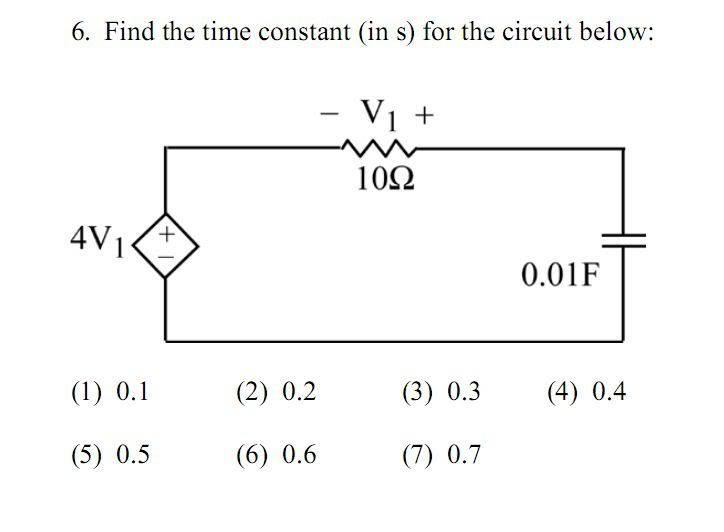# Time Constant RC circuit with dependent source

• Engineering

## Homework StatementT = R*C

## The Attempt at a Solution

I have no idea how to go about this. I know that T=R*C in a RC circuit

Mentor

## Homework StatementT = R*C

## The Attempt at a Solution

I have no idea how to go about this. I know that T=R*C in a RC circuit

That time constant is true when you have a constant voltage source and you close a switch into the RC portion of the circuit (or similar setup). This problem looks different, since voltge source is depentent on the voltage drop through the resistor (and hence dependent on the current in the circuit).

I'd start with a loop equation for the loop, using the differential equation relating Vc and Ic. Then see if solving it gives an exponential relation still, and if it does, finding the time constant that results.

Mentor
BTW, you're going to have to assume some initial condition to solve the problem, but since it's a linear circuit, the initial condition you choose shouldn't affect the time constant (at least I don't think it will).

The combination of the resistor and the dependant source can be modeled as a resistance. Find this equivalent resistance by considering the capacitor replaced by a test current. Multiply the equivalent resistance by the capacitance of C to get the time constant.

Mentor
The combination of the resistor and the dependant source can be modeled as a resistance. Find this equivalent resistance by considering the capacitor replaced by a test current. Multiply the equivalent resistance by the capacitance of C to get the time constant.

Hah! Neat trick, and very logical. I hadn't seen that before.

But the OP should do it both ways, to check his answers...CerealSteak
Trying Adjuster's trick I basically get v_in=5*v_1, My guess is that I can translate that to a R_eq of 5. But 5*0.01 = 0.05s (an order of magnitude too small).

My guess is that I'm missing something here

edit: v_1 = 10 Ohms * i_in

v_in = 5 * (10 * i_in)

R_eq = 50 Ohms

50 Ohm * 0.01 F = 0.5 s

Last edited: